Organic Analysis

# Organic Analysis

Test Description

## 60 Questions MCQ Test Mock Test Series for IIT JAM Chemistry | Organic Analysis

Organic Analysis for Chemistry 2023 is part of Mock Test Series for IIT JAM Chemistry preparation. The Organic Analysis questions and answers have been prepared according to the Chemistry exam syllabus.The Organic Analysis MCQs are made for Chemistry 2023 Exam. Find important definitions, questions, notes, meanings, examples, exercises, MCQs and online tests for Organic Analysis below.
Solutions of Organic Analysis questions in English are available as part of our Mock Test Series for IIT JAM Chemistry for Chemistry & Organic Analysis solutions in Hindi for Mock Test Series for IIT JAM Chemistry course. Download more important topics, notes, lectures and mock test series for Chemistry Exam by signing up for free. Attempt Organic Analysis | 60 questions in 180 minutes | Mock test for Chemistry preparation | Free important questions MCQ to study Mock Test Series for IIT JAM Chemistry for Chemistry Exam | Download free PDF with solutions
 1 Crore+ students have signed up on EduRev. Have you?
Organic Analysis - Question 1

### Which of the following compound is expected to show a sharp singlet for one of its protons at δ ≥ 8 ppm in 1H NMR spectrum, given that this signal remains unaffected on shaking the solution thoroughly with D2O?

Organic Analysis - Question 2

### Sometimes, the color observed in Lassaigne’s test for nitrogen is green. It is because of:

Organic Analysis - Question 3

### Arrange the following molecules, in their chemical shift value(s) of ortho hydrogen in 1H NMR: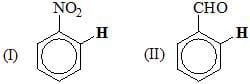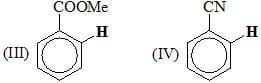Organic Analysis - Question 4

Consider the following: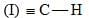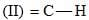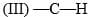Order of C-H stretching frequency in IR-spectroscopy would be:

Organic Analysis - Question 5

The major product B is: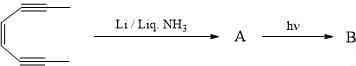Organic Analysis - Question 6

The complementary strand of DNA for the following single stranded DNA sequence: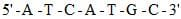is:

Organic Analysis - Question 7

The correct sequence of the amino acids present in the tripeptide given below is: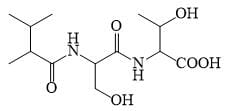Organic Analysis - Question 8

One amongst the following is an alcoholic amino acid?

Organic Analysis - Question 9

Consider the following: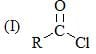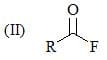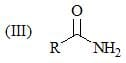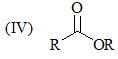Order of stretching frequency in IR-spectroscopy would be:

Organic Analysis - Question 10

For the detection of sulfur in an organic compound, Lassaigne’s extract is acidified with acetic acid. Lead acetate solution is then added to it. The black precipitate formed is due to the formation of ?

Organic Analysis - Question 11

Which of the following compound show only two signals in 1H NMR and a strong IR bond at
~1690 cm–1:

Organic Analysis - Question 12

A compound with molecular formula C5H10O does not form, 2, 4-DNP derivative. It responds positively to Iodoform test and on ozonolysis gives one mole formaldehyde. It could be:

Organic Analysis - Question 13

The correct match of the 1H NMR chemical shift d of the following species/compound is: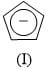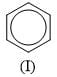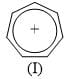Organic Analysis - Question 14

In the Lassaigne’s test for the detection of nitrogen in an organic compound, the Prussian blue colour is due to the formation of _______________:

Organic Analysis - Question 15

The correct order of 1H NMR chemical shift (δ) values for the labeled methyl groups in the following compound is: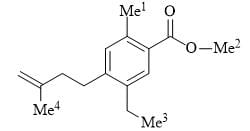Organic Analysis - Question 16

The Beckmann rearrangement of a bromo-acetophenone oxime (C8H8BrNO) gives a major product having the following 1H NMR – 9.85 (S, 1H), 7.88 (S, 1H), 7.45 (d, 1H, J=7.2), 7.17 (m, 1H, 7.12 (d, 1H, J=7.0 Hz), 2.06 (S, 3H). The structure of the product is:

Organic Analysis - Question 17

The absorption at lmax 279 nm (ε = 15) in the UV spectrum of acetone is due to:

Organic Analysis - Question 18

Match the following compounds with their respective classes: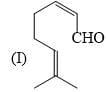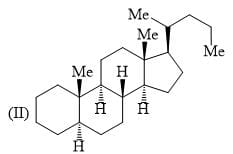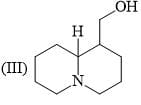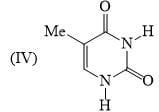Organic Analysis - Question 19

The structure of D-(+)-glucose is: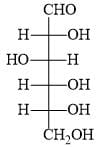The structure of L-(–)-glucose is:

Organic Analysis - Question 20

Which of the following compounds will behave as a reducing sugar in an aqueous KOH solution?

Organic Analysis - Question 21

Consider the following: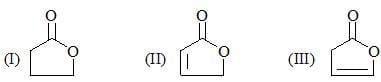Order of their stretching frequency in IR-spectroscopy would be:

Organic Analysis - Question 22

In the UV-visible absorption spectrum of an α, β-unsaturated carbonyl compound, with increasing solvent polarity:

Organic Analysis - Question 23

Among the following, the compound that displays an IR band at 2150 cm–1 is:

Organic Analysis - Question 24

The compound which shows IR frequencies at both 3314 and 2126 cm–1 is:

Organic Analysis - Question 25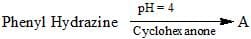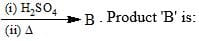Organic Analysis - Question 26

Compound I gives a strong infrared absorption at 1730 cm–1. 1H NMR spectrum indicates that it has two types of hydrogen atoms; one H atom appearing as singlet at δ = 9.7 ppm and 9H atoms appearing as a singlet at δ = 1.2 ppm. The structure of I is:

Organic Analysis - Question 27

Major product would be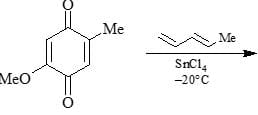Organic Analysis - Question 28

The major product of the following is: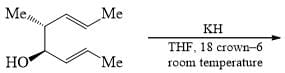Organic Analysis - Question 29

Consider the following: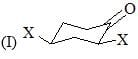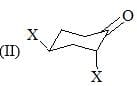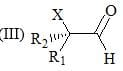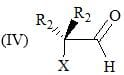Order of C-O stretching frequency in IR-spectroscopy would be:

Organic Analysis - Question 30

During the detection of sulfur in an organic compound, the violet color formed by the addition of Sodium nitroprusside to Lassaigne’s extract is due to _____________:

*Multiple options can be correct
Organic Analysis - Question 31

Lassaigne’s test for the detection of nitrogen do/does not fail in:

*Multiple options can be correct
Organic Analysis - Question 32

Which of the following compound exist(s) in Zwitter ion form:

Detailed Solution for Organic Analysis - Question 32

Amino acids and Sulfamic acids exist as Zwitter Ions. Option C is neither an amino acid nor sulfamic acid so, does not exist as Zwitter ion where as the rest of them do.

*Multiple options can be correct
Organic Analysis - Question 33

Which of the following are present in RNA?

*Multiple options can be correct
Organic Analysis - Question 34

Which of the following is/are alkaloid(s):

*Multiple options can be correct
Organic Analysis - Question 35

An organic compound having the molecular formula C10H14 exhibited three singlets in the 1H NMR spectrum. The compound(s) may be:

*Multiple options can be correct
Organic Analysis - Question 36

Which of the following molecule/s will absorb infrared radiation?

*Multiple options can be correct
Organic Analysis - Question 37

Which of the following statement(s) is correct about the Amino acids:

*Multiple options can be correct
Organic Analysis - Question 38

Identify the correct pair of statements.

(i) Alternate name of thymine is 5-methyl uracil
(ii) Arachidonic acid molecule contains less number of carbons than palmitic acid
(iii) Cellulose contains complex halices
(iv) Aquaporin is a polypeptide

Detailed Solution for Organic Analysis - Question 38

i) Thymine is a nitrogenous base which pairs with adenine by the formation of two hydrogen bond. The molecular structure includes a ring of carbon and nitrogen with nitrogen at first and third position. A methyl group is attached to fifth carbon and an oxygen atom is attached to the second carbon. It is also known as 5-methyl uracil.

iv) Aquaporin is a water transport channel present in the cell membrane which is present in the cell. It helps in the selective transport of the water molecules.

Whereas, cellulose is the polysaccharide of unbranched chain and arachidonic acid (C20​H32​O2​) has more number of the carbon atom as compared to palmitic acid (C12​H22​O2​).

So, the correct answer is option D.

*Multiple options can be correct
Organic Analysis - Question 39

Which of the following pyrimidine base(s). Find in DNA:

*Multiple options can be correct
Organic Analysis - Question 40

Out of following which is not an excitation source in IR-spectroscopy is:

*Answer can only contain numeric values
Organic Analysis - Question 41

The maximum no of dipeptides that could be obtained by reaction of phenylalanine with leucine is____?

*Answer can only contain numeric values
Organic Analysis - Question 42

Calculate the isoelectric point (pI) of lysine. Given the pKa of α-NH3 is 8.95, pKa of side chain NH3 is 10.53 and pKa of α-COOH is 2.18………………:  (up to 2 decimal place)

*Answer can only contain numeric values
Organic Analysis - Question 43

Thymine is a DNA base and has a –CH3 group at Xth position. ‘X’ is:

*Answer can only contain numeric values
Organic Analysis - Question 44

The value of (X + Y) would be if X = Total number of Monoterpenes and Y = Total number of Diterpenes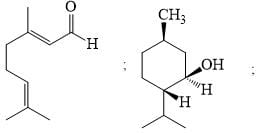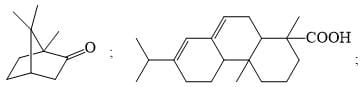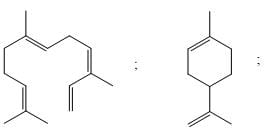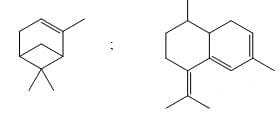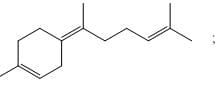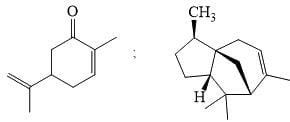*Answer can only contain numeric values
Organic Analysis - Question 45

The total number of essential amino acids are: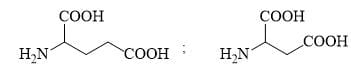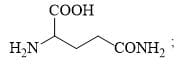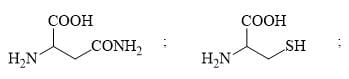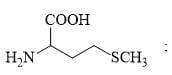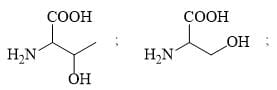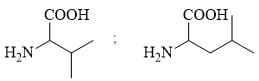*Answer can only contain numeric values
Organic Analysis - Question 46

In the Iodoform reaction of RCOCH3, ‘X’ mole of I2 and ‘Y’ mole of NaOH are used. Find the value of ‘X + Y’:

*Answer can only contain numeric values
Organic Analysis - Question 47

Number of correct statements are:

I. Tertiary amines are insoluble in HBr

II. Secondary amines are insoluble in NaOH

III. Primary amines on treatment with NaNO2/HCl produce N2 gas

IV. Generally, amino acids on treatment with Ninhydrin produce blue-voilet color

V. Fehling’s solution gives positive test for both aliphatic and aromatic aldehydes

VI. Acetic acid on treatment with FeCl3 produces blood red coloration

VII. Formic acid gives positive Tollen’s test

*Answer can only contain numeric values
Organic Analysis - Question 48

How many of the following pairs give same osazone?

Glucose-Fructose, Galactose-Talose, Altrose-Allose, Ribose-Arabinose, Idose-Gulose

*Answer can only contain numeric values
Organic Analysis - Question 49

Given the fact that 1, 3-butadiene has a UV absorption of 217 nm, the absorption wavelength (in nm) for the conjugated system shown below is……………,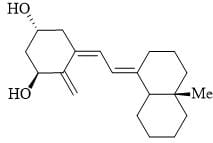(Use these absorption values for auxochromes groups:Alkyl: + 5; exo-cyclic double bond: + 5 every additional conjugated C = C : + 30)

*Answer can only contain numeric values
Organic Analysis - Question 50

How many moles of formaldehyde will be formed?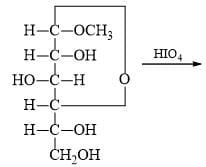*Answer can only contain numeric values
Organic Analysis - Question 51

If 500 glucose molecules are used to make starch, how many molecules of water would be produced in the condensation reactions? [Think of starch as a linear chain of glucose molecules]:

*Answer can only contain numeric values
Organic Analysis - Question 52

How many essential amino acids are there among following?

Histidine, isoleucine, leucine, lysine, methionine, phenylalanine, threonine, tryptophan, and valine

*Answer can only contain numeric values
Organic Analysis - Question 53

How many of the following statements regarding structure of proteins is/are true?
I. Primary structure helps in sequencing of amino acids and location of disulfide bonds.
II. Secondary structure sets up Protein back-bone
III. Denaturation of proteins is reversible
IV. Tertiary structure is found in globular proteins and can be revealed by X-ray crystallography
V. Keratin form derivatives of skin like hairs, feathers, claws, horns etc.
VI. Actin and Myosin are called Muscle proteins
VII. Thrombin helps in blood coagulation

*Answer can only contain numeric values
Organic Analysis - Question 54

How many of the following compounds form zwittor ions?
Glycine, Sulphanilic acid, Anthranilic acid, Lysine, Tryptophan, Leucine.

*Answer can only contain numeric values
Organic Analysis - Question 55

The total number of compound for which δAB in 1H NMR spectroscopy: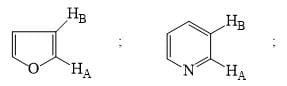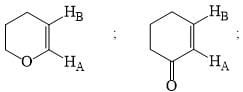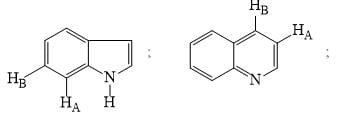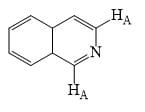*Answer can only contain numeric values
Organic Analysis - Question 56

The total number of carbonyl compounds which have higher IR stretching frequency than acetone: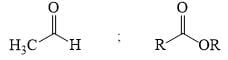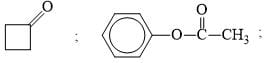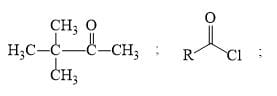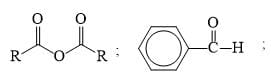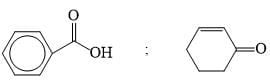*Answer can only contain numeric values
Organic Analysis - Question 57

The number of reducing sugars among the following is……………..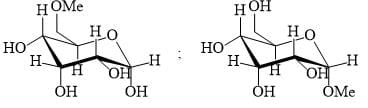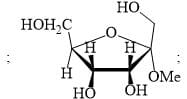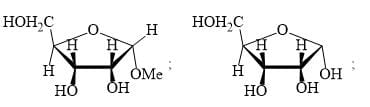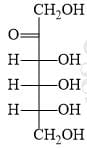*Answer can only contain numeric values
Organic Analysis - Question 58

Number of 1H NMR signals in Phenanthracene?

*Answer can only contain numeric values
Organic Analysis - Question 59

If two signal differ by 1.5 ppm in 300 MHz spectrophotometer, how do they differ (in ppm) in 100 MHz?

*Answer can only contain numeric values
Organic Analysis - Question 60

How many different dipeptides could be made with 20 types of amino acids?

## Mock Test Series for IIT JAM Chemistry

2 docs|25 tests
 Use Code STAYHOME200 and get INR 200 additional OFF Use Coupon Code